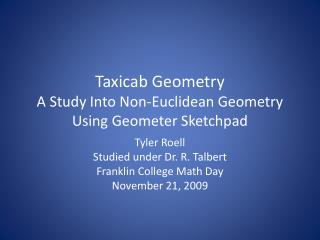DownloadDownload PresentationTaxicab Geometry A Study Into Non-Euclidean Geometry Using Geometer Sketchpad

# Taxicab Geometry A Study Into Non-Euclidean Geometry Using Geometer Sketchpad

Download Presentation## Taxicab Geometry A Study Into Non-Euclidean Geometry Using Geometer Sketchpad

- - - - - - - - - - - - - - - - - - - - - - - - - - - E N D - - - - - - - - - - - - - - - - - - - - - - - - - - -
##### Presentation Transcript

1. Taxicab Geometry A Study Into Non-Euclidean GeometryUsing Geometer Sketchpad Tyler Roell Studied under Dr. R. Talbert Franklin College Math Day November 21, 2009

2. The Beginning of Taxicab Geometry? Hermann Minkowski (1864-1909) Teacher of Einstein Also known as Manhattan Distance Karl Menger (1952)

3. What is Taxicab Geometry? Assumptions made of Taxicab Geometry One Main Difference Dt=|x1-x2|+|y1-y2|

4. Differences Dt=|x1-x2|+|y1-y2| De= Crows Fly Vs. Urban Geography Dt=|3-1|+|1-4|=5

5. Redistricting Problem Say you have three schools, K,N, and M. Using the Taxicab Distance, where should the school districts be drawn to keep all the districts the same size in area?

6. Geometric Figures Circles The set of all points equidistant from a point p with radius r

7. Geometric Figures Parabolas set of all points that are the same distance from a fixed line and a fixed point not on the line

8. Geometric Figures Parabolas set of all points that are the same distance from a fixed line and a fixed point not on the line

9. Bisectors • In Taxicab In Euclidean

10. Now back to the problem What should be done first? What figures should be used?

11. Construct circles with the same radius

12. Construct the bisector between K and N

13. Bisector between N and M

14. Bisector between K and M

15. The Solution

16. Further Application Ellipses The set of all points where the sum of the distances from any point on the curve to the two fixed points remain a constant

17. Applications for Teaching • Easier way to measure distance • Applicable to the Urban Setting • Uses technology in the Classroom

18. Book Used • Eugene F. Krause Taxicab Geometry: An Adventure in Non-Euclidean Geometry